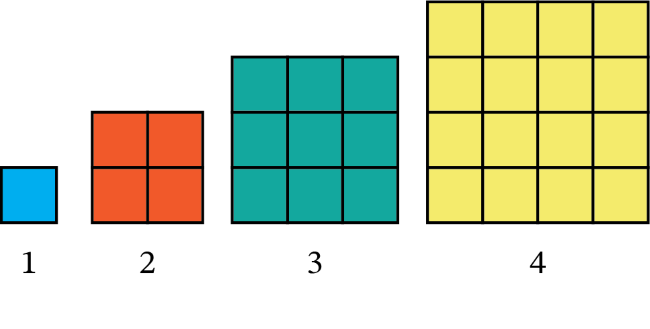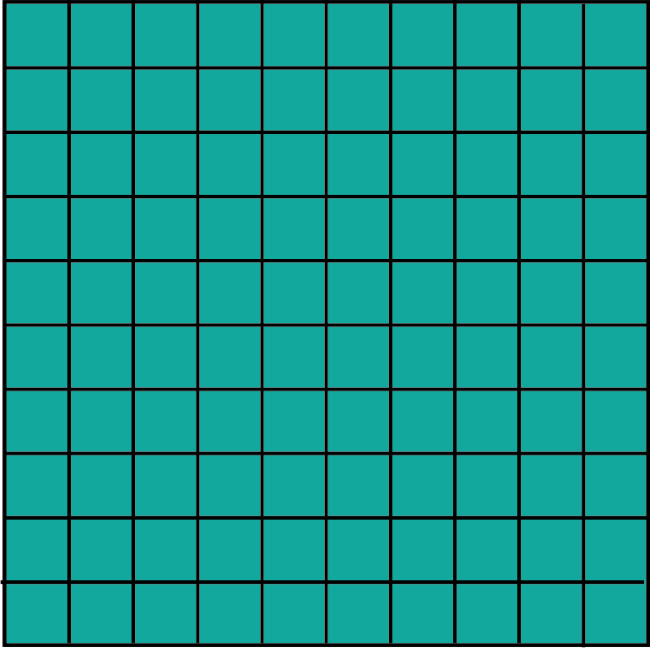# Perfect Squares and Square RootsHello, in this video, we will explore how to simplify square roots and find perfect squares.

The symbol in math that we use to represent square roots is called a radical, and it looks like this: $$\sqrt{}$$. The number that appears under the radical is called the radicand. For example, in this expression, $$\sqrt{20}$$, which we read as, “the square root of twenty,” 20 is the radicand. Just like the fact that 20 is the same as $$\frac{20}{1}$$, or $$20^{1}$$, but we don’t write the 1, because it is a given, in square roots there is something similar. There is a given 2 in the bent arm of the radical that we do not normally write when we are looking for a square root, $$\sqrt{}$$. This number is called the index and it determines what root of the radicand we are trying to find. For example, this: $$\sqrt{20}$$, is asking for the square root, or the second root of 20 and this: $$\sqrt{125}$$, which we read as “the cube root of one hundred twenty-five,” is asking us to find the third root of 125.

I want you to practice this on your own. Pause the video and label the different parts of this expression: $$\sqrt{200}$$.

Think you’ve got it? The root symbol is the radical. The index is 4 because it’s the number in the bent arm of the radical. And the radicand is the number under the radical symbol, so in this case, 200.

$$\sqrt{}$$ – radical

4 – index

Great work!

Now let’s talk about how we find the square root of a number. To do this, we ask ourselves, “what number multiplied by itself will give us the radicand?” For example, what is the square root of 25: $$\sqrt{25}$$? The factors of 25 are $$5\times 5$$, which means that the square root of 25 is 5. This also happens to be what we call a perfect square. A perfect square is when we are simplifying a square root and nothing remains under the radical.

What are the square roots of 36, 81, and 144? Pause the video and try these on your own. When you’re finished, we’ll look over them together.

$$\sqrt{36}=6, \text{ because}\text{ }6\times 6=36$$

$$\sqrt{81}=9, \text{ because}\text{ }9\times 9=81$$

$$\sqrt{144}=12, \text{ because}\text{ }12\times 12=144$$

We get these nice, pretty numbers because we are taking the square roots of perfect squares. But what if we aren’t given a perfect square? Well, then we will have to simplify the square root.

## Simplifying Square Roots

When simplifying a square root, we will get all the perfect squares out from under the radical and whatever is remaining from the factors of the radicand stays under the radical. Let’s look at an example.

Simplify the square root of 40: $$\sqrt{40}$$.

We will start by finding the factors of 40, which are $${2}\times {2}\times {2}\times{5}$$.

Since $$2\times{2}$$ creates a perfect square, we can bring the 2 to the front and what remains under the radical is $$2\times{5}$$.

There are no more perfect squares to take out, so we simply multiply these numbers together to get our new radicand.

Therefore, the square root of 40 is equal to 2 square root of 10: $$\sqrt{40}$$ = $$2\sqrt{10}$$.

Now I want you to try one. Simplify the square root of 96: 96. Pause the video here and simplify. When you’re done, we’ll take a look at it together.

Think you’ve got it? First, we need to find the factors of 96.

$$96 = 2 \times {2}\times {2}\times {2} \times {2}\times{ 3}$$

It is easy to see the perfect squares when the factors are written in this form. We can pull out two sets of 2 from our radicand, which will leave us with $$2\times{2}=4$$ in front of our new radical. We still have a 2 and a 3 from our factor list, so we multiply these numbers together to get 6, and this is our new radicand. Therefore, the most simplified form is:

$$\sqrt{96}= 4\sqrt{6}$$

I hope this video on square roots and perfect squares was helpful. Thanks for watching, and happy studying!

### How do I find a square root?

#### A

The square root is the inverse of squaring a number. When we square a number we multiply the number by itself. 4 squared is $$4\times4$$, which equals 16. 16 is a perfect square, and 4 is the square root of 16. Not all numbers will have a nice whole number square root. For example, a number like 50 is not a perfect square because it does not have an integer square root. There is no way to multiply an integer by itself to create a product of 50. 50 does not have an integer square root, but 49 does. The square root of 49 is 7 because $$7\times7$$ equals 49. 49 is considered the perfect square, and 7 is considered the square root. The radical symbol is used to notate square roots. For example, the square root of 49 is expressed as $$\sqrt{49}$$:$$\sqrt{49}=7$$. This is read as “the square root of 49 equals 7”.

### What are the whole number square roots from 1 to 20?

#### A

The whole number square roots from 1 to 20 are $$\sqrt1=1$$,$$\sqrt4=2$$,$$\sqrt9=3$$, and $$\sqrt{16}=4$$.

Not all numbers will have whole number square roots. For example, 1 has a square root because 1 can be expressed as the product of two equal integers, 1ｘ1. 4 has a whole number square root because it can be expressed as the product of 2ｘ2. However, notice how 2 and 3 will not have whole number square roots. 2 cannot be expressed as an integer multiplied by itself. Similarly, 3 cannot be expressed as an integer multiplied by itself.

### What are numbers with integer square roots?

#### A

Numbers with integer square roots are called perfect squares. For example, 64 is a number with an integer square root. 64 is the product of $$8\times8$$. When a number can be created by multiplying an integer by itself, it is called a perfect square. Examples of numbers with integer square roots are 1, 4, 9, 16, and 25. All of these numbers have integer square roots. 25 is a perfect square which is the product of $$5\times5$$. This means that 5 is the square root of 25.

Not all numbers will have integer square roots. For example, 5 does not have an integer square root. No integer times itself has a product of 5. We can get close: $$1\times=1$$, $$2\times2=4$$, and $$3\times3=9$$, but 5 is not a perfect square, so it does not have an integer square root.

### What are the first 20 perfect squares?

#### A

The first 20 perfect squares are 1, 4, 9, 16, 25, 36, 49, 64, 81, 100, 121, 144, 169, 196, 225, 256, 289, 324, 361, and 400. Perfect squares are found by squaring an integer. $$1\times1=1$$,$$2\times2=4$$,$$3\times3=9$$,$$4\times4=16$$… and so on. Not every number will be a perfect square. Perfect squares can be thought of as literal squares. A square is created using two equal integers as side lengths. This means that the result, or product, will be a perfect square.### What are the perfect squares from 1 to 100?

#### A

There are only ten perfect squares from 1 to 100. 1, 4, 9, 16, 25, 36, 49, 64, 81, and 100. These perfect squares are the result of multiplying a number by itself. For example, 1 is a perfect square because $$1\times1=1$$. 4 is a perfect square because $$2\times2=4$$. 9 is a perfect square because $$3\times3=9$$. Not all numbers are perfect squares. It can be helpful to think of perfect squares as actual squares with a length and a width. For example, 100 is a perfect square built from side lengths of 10 and 10. $$10\times10=100$$, therefore 100 is a perfect square.### How do you determine perfect squares?

#### A

Numbers that are considered perfect squares are the result of multiplying an integer by itself. For example, 25 is a perfect square because it is the product of $$5\times5$$. A number multiplied by itself creates a perfect square.

In order to determine if a number is a perfect square, you need to identify if it is the product of an integer squared. If the number can be formed by squaring an integer, then it is a perfect square. For example, 33 is not a perfect square because there is no way to square an integer number and have the product be 33. We can be sure this is true since $$5\times5=25$$ and $$6\times6=36$$. Because 33 is between 25 and 36, its square root must be between $$\sqrt{25}=5$$ and $$\sqrt{36}=6$$. Since there is no integer between 5 and 6, 33 cannot have an integer square root. However, 36 is a perfect square because it is the result of squaring 6. $$6^2=36$$, so 36 is considered a perfect square.

### Why are there no perfect squares between 144 and 169?

#### A

Perfect squares are the result of squaring a number. For example, 81 is a perfect square because it is the result of squaring 9: $$9\times9=81$$. 144 is a perfect square because it is the result of squaring 12: $$12\times12=144$$. 169 is a perfect square because it is the result of squaring 13: $$13\times13=169$$. Notice how there are no perfect squares between 144 and 169. If a number is between 144 and 169, its square root must be between $$\sqrt{144}=12$$ and $$\sqrt{169}=13$$. Since there is no integer between 12 and 13, there are no perfect squares between 144 and 169.

We cannot create a perfect square by multiplying something like $$11.5\times11.5$$ because it is not the product of two equal integers. The square roots of perfect squares need to be integers.

## Practice Questions

Question #1:

Which set of numbers contains all perfect squares?

33, 99, 55, 66

33, 99, 55, 66

36, 9, 25, 100

81, 36, 25, 41

The correct answer is 36, 9, 25, 100. Thirty-six is a perfect square composed of $$6×6$$, nine is a perfect square composed of $$3×3$$, twenty-five is a perfect square composed of $$5×5$$, and one hundred is a perfect square composed of $$10×10$$.

Question #2:

Which pair shows a correct match between the perfect square and its whole number square root?

$$\sqrt{144}=12$$
$$\sqrt{36}=2$$
$$\sqrt{25}=4$$
$$\sqrt{100}=11$$

The correct answer is $$\sqrt{144}=12$$. One hundred forty-four is a perfect square composed of $$12×12$$. Thirty-six is a perfect square, but it is not composed of $$2×2$$. It is instead composed of $$6×6$$. Twenty-five is a perfect square, but it is not composed of $$4×4$$. It is instead composed of $$5×5$$. One hundred is a perfect square, but it is not composed of $$11×11$$. It is instead composed of $$10×10$$.

Question #3:

What is the square root of 49?

6

4

5

7

The correct answer is 7. The square root of 49 is 7, because $$7×7=49$$. This also means that 49 is a perfect square.

Question #4:

Which value is NOT a perfect square?

81

100

64

99

The correct answer is 99. Because there is no whole number that can be multiplied by itself to equal 99, it is not a perfect square. Eighty-one is a perfect square composed of $$9×9$$, one hundred is a perfect square composed of $$10×10$$, and sixty-four is a perfect square composed of $$8×8$$.

Question #5:

Which pair shows an incorrect match between the perfect square and its whole number square root?

$$\sqrt{49}$$ and $$7$$

$$\sqrt{4}$$ and $$2$$

$$\sqrt{64}$$ and $$8$$

$$\sqrt{9}$$ and $$6$$

The correct answer is $$\sqrt{9}$$ and $$6$$. The square root of 9 is a perfect square, but it is composed of $$3×3$$, not $$6×6$$.